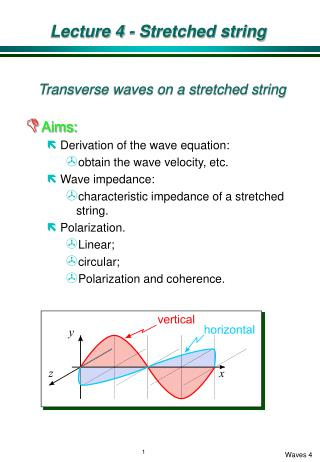DownloadDownload PresentationLecture 4 - Stretched string

# Lecture 4 - Stretched string

Télécharger la présentation## Lecture 4 - Stretched string

- - - - - - - - - - - - - - - - - - - - - - - - - - - E N D - - - - - - - - - - - - - - - - - - - - - - - - - - -
##### Presentation Transcript

1. Lecture 4 - Stretched string Transverse waves on a stretched string • Aims: • Derivation of the wave equation: • obtain the wave velocity, etc. • Wave impedance: • characteristic impedance of a stretched string. • Polarization. • Linear; • circular; • Polarization and coherence.

2. Derivation of the wave equation • General approach: • Consider a small segment; • Find the difference in forces on the two ends; • Find the element’s response to this imbalance. • We need the following useful result: • easy to see graphically: • Waves on a string: • Neglect extension of the string i.e. tension is constant everywhere and unaltered by the wave. • Displacements are small so • Y<<l. • ¶Y/¶x and q are << 1. • Neglect gravity

3. Stretched string • Forces on an element of the string: • Net force in the y-direction isButso • Net transverse force = • To apply Newton’s 2nd law we need mass and acceleration. These are:mass of element =acceleration (transverse) =

4. Derivation of wave equation • Newton’s second law: • The wave velocity is not the transverse velocity of the string, which is • Two Polarization's for a transverse wave: • Horizontal (z) ; Vertical (y). acceleration mass force

5. Wave impedance • The general concept: • Applying a force to a wave medium results in a response and we can therefore define an impedance = applied force/velocity response. • Expect the impedance to be real (force and velocity are in-phase) - since energy fed into the medium propagates (without loss) away from the source of the excitation. • Imaginary impedance - no energy can be transported (examples later: waveguide below cut-off). • Complex impedance - lossy medium. • Characteristic impedance: • Transverse driving force: • Transverse velocity:

6. Impedance of a stretched string • Impedance • For a wave in +ve x-direction, recall • Impedance (for wave in +ve x-direction) • Wave in -ve x-direction, Z has the opposite sign: • Note that the impedance is real. i.e. the medium is lossless (in this idealised picture).

7. Energy in a travelling wave • Energy is both kinetic and potential. • Kinetic energy associated with the velocity of elements on the string. • Potential energy associated with the elastic energy of stretching during the motion. • Potential energy density (P.E./unit length) • Calculate the work done increasing the length of segment Dx against a constant tension T. • Increase in length of segment is

8. Energy density • Increase in P.E (= force x extension) • Potential energy density • Kinetic energy density • K.E. of length Dx • Density • Note: instantaneous KE and PE are equal since • Total energy density • Sum of KE and PE extension force 1/2 v2 m

9. Energy: harmonic wave • Average energy density(section 1.1.3) • average energy density is • consider each element, length Dx, as an oscillator with energy • In time Dt we excite a length Dx=vDt. So the energy input is • Since Z=rv, • Mean power